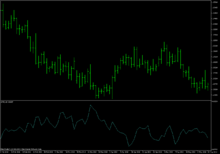# Average true range

Average true range (ATR) is a technical analysis volatility indicator originally developed by J. Welles Wilder, Jr. for commodities. The indicator does not provide an indication of price trend, simply the degree of price volatility. The average true range is an N-day smoothed moving average (SMMA) of the true range values. Wilder recommended a 14-period smoothing.

## CalculationMetaTrader EUR/USD chart showing ATR indicator (cyan line) with period 14.

The range of a day's trading is simply. The true range extends it to yesterday's closing price if it was outside of today's range.The true range is the largest of the:

• Most recent period's high minus the most recent period's low
• Absolute value of the most recent period's high minus the previous close
• Absolute value of the most recent period's low minus the previous close

The ATR at the moment of time t is calculated using the following formula: (This is one form of an exponential moving average)The first ATR value is calculated using the arithmetic mean formula:The idea of ranges is that they show the commitment or enthusiasm of traders. Large or increasing ranges suggest traders prepared to continue to bid up or sell down a stock through the course of the day. Decreasing range suggests waning interest.

## Applicability to futures contracts vs. stocks

Since true range and ATR are calculated by subtracting prices, the volatility they compute does not change when historical prices are back-adjusted by adding or subtracting a constant to every price. Back-adjustments are often employed when splicing together individual monthly futures contracts to form a continuous futures contract spanning a long period of time. However the standard procedures used to compute volatility of stock prices, such as the standard deviation of logarithmic price ratios, are not invariant (to addition of a constant). Thus futures traders and analysts typically use one method (ATR) to calculate volatility, while stock traders and analysts typically use another (SD of log price ratios).

## Use in position size calculation

Apart from being a trend strength gauge, ATR serves as an element of position sizing in financial trading. Current ATR value (or a multiple of it) can be used as the size of the potential adverse movement (stop-loss distance) when calculating the trade volume based on trader's risk tolerance. In this case, ATR provides a self-adjusting risk limit dependent on the market volatility for strategies without a fixed stop-loss placement. A less volatile market has a larger trading position in comparison to a more volatile market in a portfolio.

## References

1. J. Welles Wilder, Jr. (June 1978). New Concepts in Technical Trading Systems. Greensboro, NC: Trend Research. ISBN 978-0-89459-027-6.
2. ATR Definition - investopedia.com
3. Joel G. Siegel (2000). International encyclopedia of technical analysis. Global Professional Publishing. p. 341. ISBN 978-1-888998-88-7.
4. This is by his reckoning of SMMA periods, meaning an α=1/14.
5. Average True Range calculation
6. http://www.earnforex.com/blog/position-sizing-rules/#atr-based-position-sizing